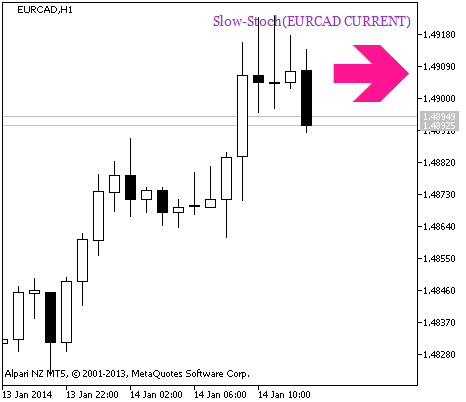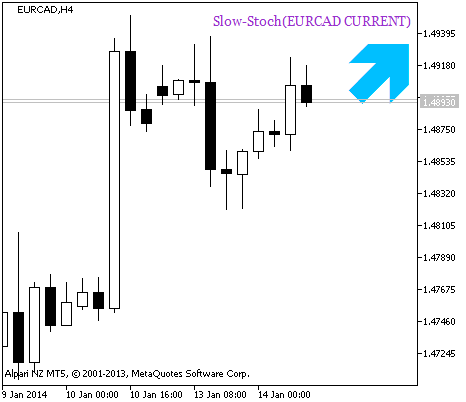887

2014.06.16 09:05

2016.11.22 07:33

Slow-Stoch_HTF_Signal 在选择的柱线上显示基于 Slow-Stoch 指标数据的趋势方向信息作为图形对象，并生成警报或语音信号，以及当交易信号出现，发出推送通知到智能手机。

`input uint SignalBar=0;   // 接受信号的柱线号码 (0 - 当前柱线)`

1. 指标 Slow-Stoch 的输入参数:
```input string Symbol_="";                        // 品名
input ENUM_TIMEFRAMES Timeframe=PERIOD_H6;      // 指标计算的时间帧

input uint KPeriod=5;
input uint DPeriod=3;
input uint Slowing=3;
input ENUM_MA_METHOD STO_Method=MODE_SMA;
input ENUM_STO_PRICE Price_field=STO_LOWHIGH;
input Smooth_Method XMA_Method=MODE_JJMA;       // 平均方法
input uint XLength=5;                           // 平滑深度
input int XPhase=15;                            // 平滑参数
//---- 对于 JJMA 它的变化范围 -100 ... +100 并且影响平滑中间处理的品质;
//---- 对于 VIDIA 它是 CMO 周期, 对于 AMA 它是慢速移动平均周期```
2. 指标 Slow-StochI_HTF_Signal 所需的可视化输入参数:
```//---- 可视化输入参数
input uint SignalBar=0;                                 // 信号生成柱线号 (0 -当前柱线)
input string Symbols_Sirname=INDICATOR_NAME"_Label_";   // 指标标签名称
input color UpSymol_Color=Lime;                         // 上涨品种颜色
input color DnSymol_Color=Magenta;                      // 下跌品种颜色
input color IndName_Color=DarkOrchid;                   // 指标名称颜色
input uint Symbols_Size=60;                             // 指标符号大小
input uint Font_Size=10;                                // 指标名字号
input int X_1=5;                                        // 名称水平平移
input int Y_1=-15;                                      // 名称垂直平移
input bool ShowIndName=true;                            // 显示指标名称
input ENUM_BASE_CORNER  WhatCorner=CORNER_RIGHT_UPPER;  // 边角位置
input uint X_=0;                                        // 水平平移
input uint Y_=20;                                       // 垂直平移
```
3. 指标 Slow-StochI_HTF_Signal 所需的生成警报和声音信号输入参数:
```//---- 警报设置
input bool Push=true;                        // 允许推送通知Exp_Slow-StochMultiSlow-Stochx7SignalrvmGann_sv8ADXCloud_HTF